# JEE Main 2020 Physics Paper With Solutions Shift 2 Sept 5

JEE Main 2020 Paper with Solutions Physics - Shift 2 will help the students to prepare efficiently for the JEE Main exams by understanding the difficulty level of the question paper. Practising JEE Main previous year question papers with answer keys will help the aspirants to score more marks in the IIT JEE Examinations. This set of solutions is designed to help the candidates in achieving full marks and also to overcome their academic weaknesses by identifying gaps in exam-taking skills.

### September 5 Shift 2 - Physics

Question 1: A ring is hung on a nail. It can oscillate, without slipping or sliding

(i) in its plane with a time period T1 and,

(ii) back and forth in a direction perpendicular to its plane, with a period T2.

The ratio T1 / T2 will be:

1. 1) 3 / √2
2. 2) √2 / 3
3. 3) 2 / √3
4. 4) 2 / 3

Solution: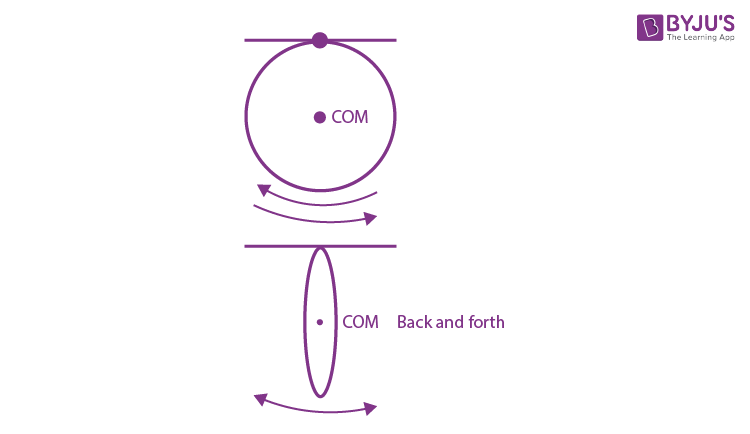$$T_{1}=2\pi \sqrt{\frac{(mR^{2}+mR^{2})}{mgR}}\\ T_{1}=2\pi \sqrt{\frac{2R}{g}}\\ T_{2}=2\pi \sqrt{\frac{I}{mgL_{cm}}}\\ T_{2}=2\pi \sqrt{\frac{\frac{3mR^{2}}{2}}{mgR}}=2\pi\sqrt{\frac{3R}{2g}\\ }\\ \frac{T_{1}}{T_{2}}=\sqrt{\frac{4}{3}}=\frac{2}{\sqrt{3}}$$

Question 2: The correct match between the entries in column I and column II are:

 I II Radiation Wavelength (a) Microwave (i) 100 m (b) Gamma rays (ii) 10–15 m (c) A.M. radio waves (iii) 10–10 m (d) X-rays (iv) 10–3 m

1. 1) (a) - (ii), (b)-(i), (c)-(iv), (d)-(iii)
2. 2) (a)-(iii), (b)-(ii), (c)-(i), (d)-(iv)
3. 3) (a)-(iv), (b)-(ii), (c)-(i), (d)-(iii)
4. 4) (a)-(i),(b)-(iii), (c)-(iv), (d)-(ii)

Solution:

By theory

Question 3: In an experiment to verify Stokes law, a small spherical ball of radius r and density falls under gravity through a distance h in the air before entering a tank of water. If the terminal velocity of the ball inside water is the same as its velocity just before entering the water surface, then the value of h is proportional to (ignore viscosity of air)

1. 1) r4
2. 2) r
3. 3) r3
4. 4) r2

Solution: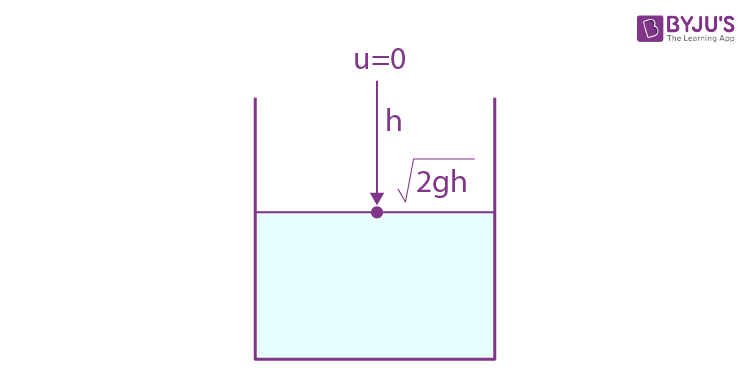VT = √2gh

[(2 / 9) r2] [(ρ - σ) / η] = √2gh

r2 ∝ √h ⇒ r4 ∝ h

h ∝ r4

Question 4: Ten charges are placed on the circumference of a circle of radius R with constant angular separation between successive charges. Alternate charges 1, 3, 5, 7, 9 have charge (+q) each, while 2, 4, 6, 8, 10 have charge (–q) each. The potential V and the electric field E at the centre of the circle are respectively: (Take V= 0 at infinity)

1. 1) V = 0; E = 0
2. 2) V = 10q / [4πε0R]; E = 10q / [4πε0R2]
3. 3) V = 0; E = 10q / [4πε0R2]
4. 4) V = 10q / [4πε0R]; E = 0

Solution: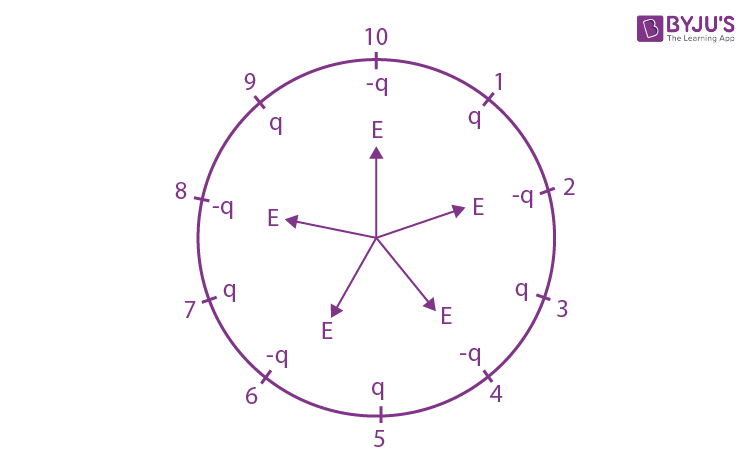vnet = 5 (kq / R) + (5k [-q]) / R

vnet = 0 [Qnet = 0]

Enet = 0 by symmetry

Question 5: A spaceship in space sweeps stationary interplanetary dust. As a result, its mass increases at a rate [dM (t)] / dt = bv2 (t), where v(t) is its instantaneous velocity. The instantaneous acceleration of the satellite is:

1. 1) –bv3(t)
2. 2) –bv3 / M(t)
3. 3) –2bv3 / M(t)
4. 4) –bv3 / 2M(t)

Solution:

[dM (t)] / dt = bv2

in free space

no external force

so there in only thrust force on rocket

fin = [dM / dt] (Vrel)

Ma = [– bv2 / (t)] v

a = –bv3 / M(t)

Question 6: Two different wires having lengths L1 and L2, and respective temperature coefficient of linear expansion ɑ1 and ɑ2, are joined end-to-end. Then the effective temperature coefficient of linear expansion is:

1. 1) [ɑ1L1 + ɑ2L2] / [L1 + L2]
2. 2) 2 √ ɑ1ɑ2
3. 3) 4 [(ɑ1ɑ2) / [ɑ1 + ɑ2])] [(L2L1 / (L2 + L1)2]
4. 4) [ɑ1 + ɑ2] / 2

Solution: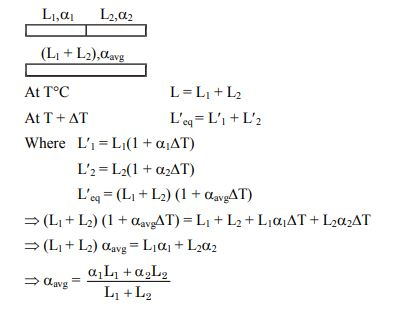Question 7: In the circuit, given in the figure currents in different branches and the value of one resistor are shown. Then potential at point B with respect to the point A is: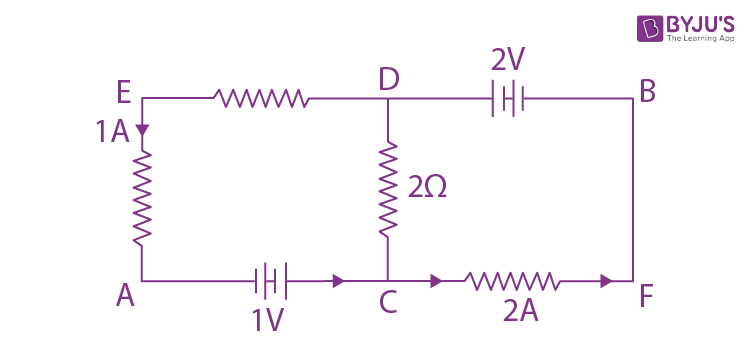1. 1) +2 V
2. 2) –2 V
3. 3) +1 V
4. 4) –1 V

Solution: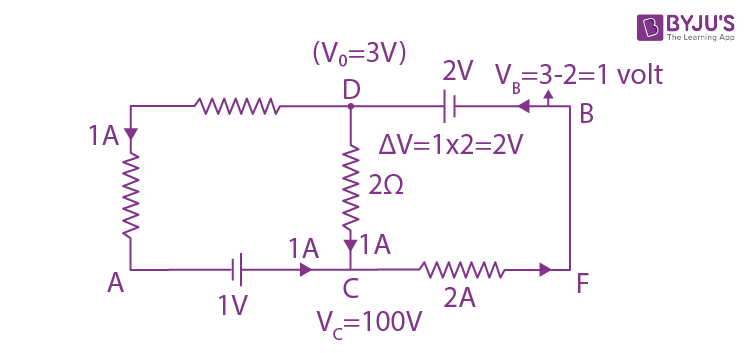Let VA = 0

Applying KVL from A to B via ACDB,

VA + 1 + 2 - 2 = VB

VB = 1V

Question 8: The velocity (v) and time (t) graph of a body in a straight line motion is shown in the figure. The point S is at 4.333 seconds. The total distance covered by the body in 6 s is: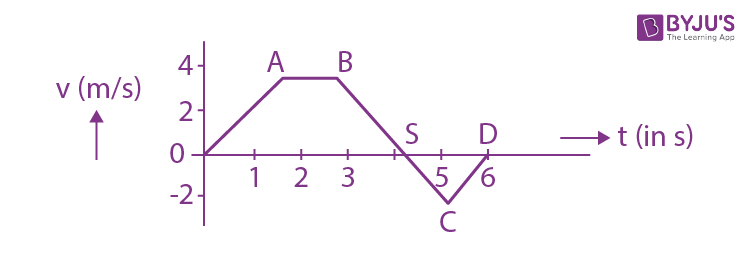1. 1) 37 / 3 m
2. 2) 49 / 4 m
3. 3) 12 m
4. 4) 11 m

Solution: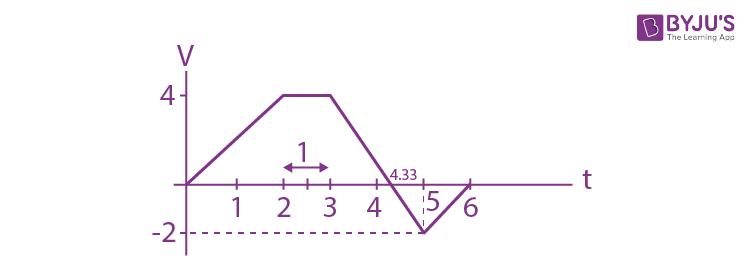Distance = area under graph

= (1 / 2) (4) [(13 / 3) + 1] + [(1 / 2) (6 - [13 / 3]) × 2]

= 2 × (16 / 3) + [5 / 3]

= (32 / 3) + (5 / 3) = [37 / 3] m

Question 9: An infinitely long straight wire carrying current I, one side opened rectangular loop and a conductor C with a sliding connector are located in the same plane, as shown in the figure. The connector has length l and resistance R. It slides to the right with a velocity v. The resistance of the conductor and the self-inductance of the loop are negligible. The induced current in the loop, as a function of separation r, between the connector and the straight wire is: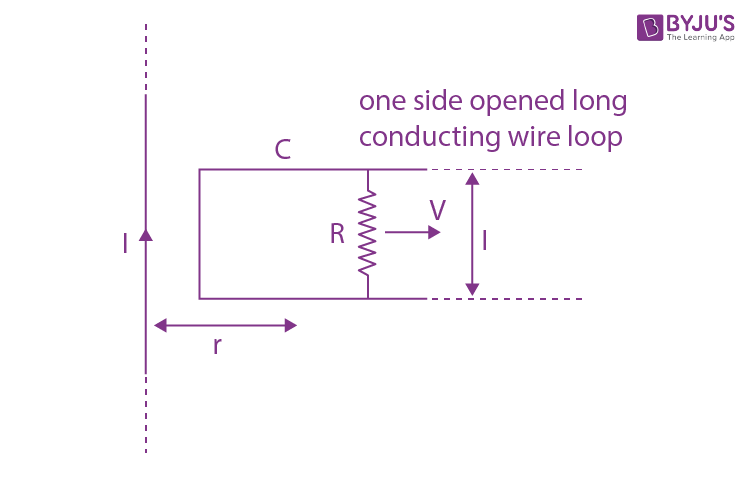1. 1) [μ0 / 2π] [Ivl /Rr]
2. 2) [μ0 / π] [Ivl /Rr]
3. 3) [2μ0 / π] [Ivl /Rr]
4. 4) [μ0 / 4π] [Ivl /Rr]

Solution: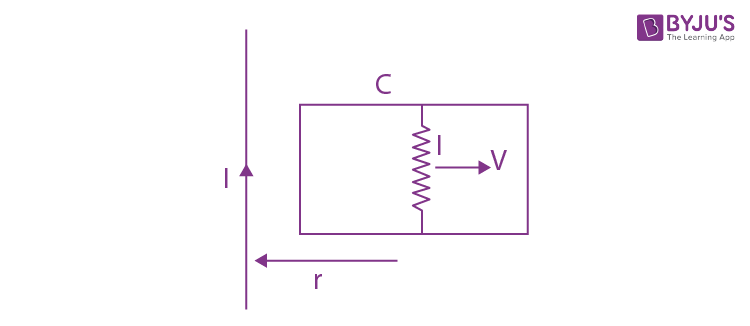B = [μ0I / 2πr]

Induced emf e = Bvl

= [μ0I / 2πr] * V . l

= [μ0Ivl / 2πr]

Induced current i = e / R = [μ0 / 2π] [Ivl /Rr]

Question 10: Two Zener diodes (A and B) having breakdown voltages of 6 V and 4 V respectively, are connected as shown in the circuit below. The output voltage VO variation with input voltage linearly increasing with time is given by (Vinput = 0 V at t = 0) (figures are qualitative)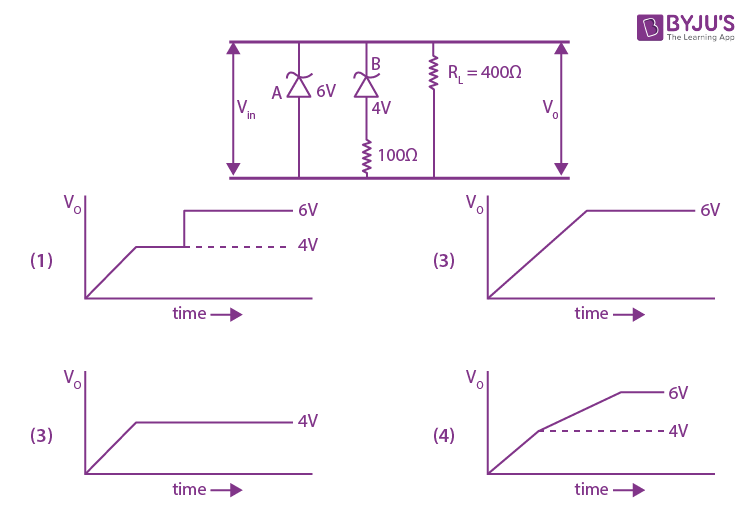Solution:

t = 0

Vi = 0

Vi ∝ t

∵ Zenerdiode maintains constant breakdown voltage.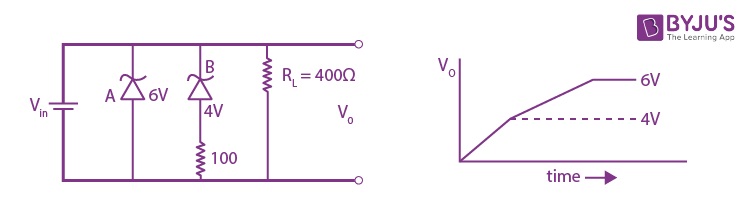Question 11: In an adiabatic process, the density of a diatomic gas becomes 32 times its initial value. The final pressure of the gas is found to be n times the initial pressure. The value of n is:

1. 1) 32
2. 2) 1 / 32
3. 3) 326
4. 4) 128

Solution:

PVγ = constant

p (⍴) = constant

p11) = p22) γ = 7 / 5 for diatomic

p0ρ0-7/5 = (np0) (32ρ0)-7/5

n = (25)7/5 = 27 = 128

Question 12: A galvanometer is used in the laboratory for detecting the null point in electrical experiments. If on passing a current of 6 mA it produces a deflection of 2°, its figure of merit is close to:

1. 1) 6 × 10–3 A/div.
2. 2) 3 × 10–3 A/div.
3. 3) 666° A/div.
4. 4) 333° A/div.

Solution:

Figure of merit = I / θ ⇒ A/div.

= [6 × 10-3] / 2

= 3 × 10–3 A/div.

Question 13: In the circuit shown, charge on the 5μF capacitor is: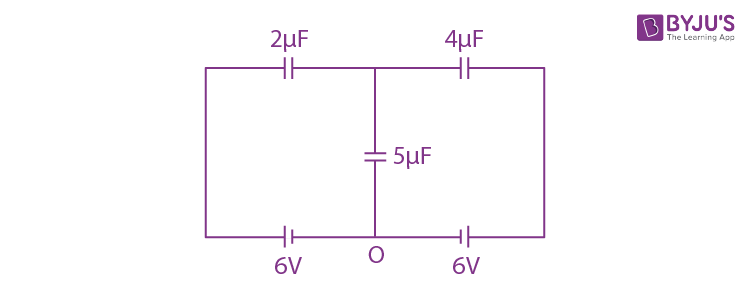1. 1) 5.45 μc
2. 2) 18.00 μc
3. 3) 10.90 μc
4. 4) 16.36 μc

Solution: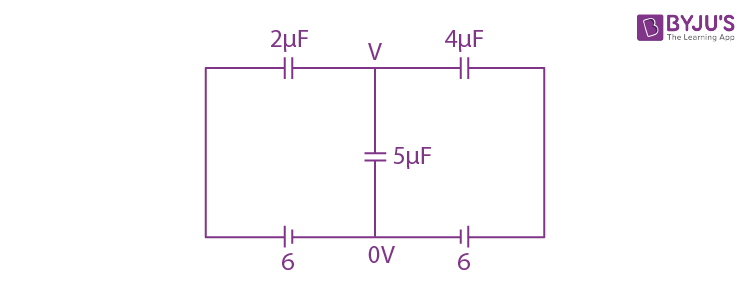(V – 6) × 2 + (V – 0) × 5 + (V – 6) 4 = 0

2V – 12 + 5V + 4V – 24 = 0

11V = 36

V = 36 / 11

q = CV = 5 × (36 / 11) = 16.36 μC

Question 14: A parallel plate capacitor has a plate of length ‘l’, width ‘w’ and separation of plates is ‘d’. It is connected to a battery of emf V. A dielectric slab of the same thickness ‘d’ and of dielectric constant k = 4 is being inserted between the plates of the capacitor. At what length of the slab inside plates, will the energy stored in the capacitor be two times the initial energy stored?

1. 1) 2l / 3
2. 2) l / 2
3. 3) l / 4
4. 4) l / 3

Solution: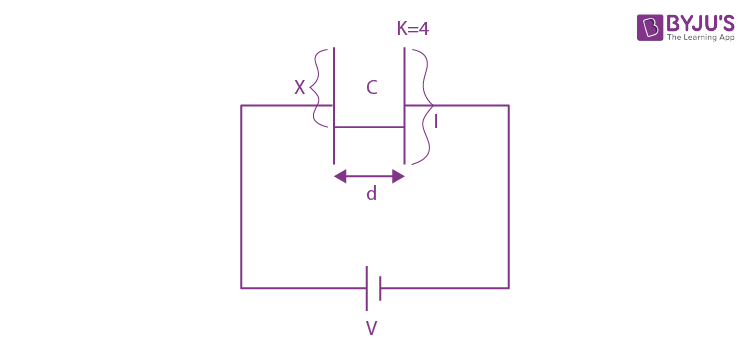area of plate = lw

C = ε0A / d = ε0lw / d

U1 = (1 / 2) cv2 = [(1 / 2) ε0lw / d] v2

Ceq = C1 + C2

When x length of the slab is inside the capacitor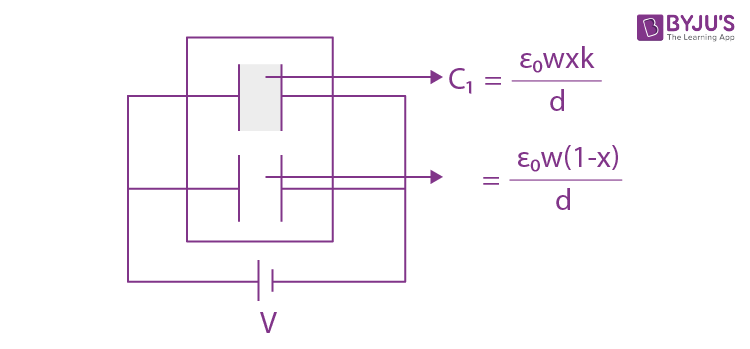Ceq = [ε0wxk / d] + [ε0w (l - x) / d]

Ceq = [ε0w / d] [kx + l - x]

Uf = (1 / 2) Ceq V2

Uf = 2Ui ⇒ (1 / 2) (ε0w / d) [kx + l - x] v2 = 2 * (1 / 2) (ε0w / d) v2

kx + l – x = 2l

4x – x = l

3x = l

x = l / 3

Question 15: A radioactive nucleus decays by two different processes. The half-life for the first process is 10 s and that for the second is 100 s. The effective half-life of the nucleus is close to:

1. 1) 55 sec.
2. 2) 6 sec.
3. 3) 12 sec.
4. 4) 9 sec.

Solution:

T1 = 10 sec; λ1 = [ln 2 / T1]

T2 = 100 sec; λ2 = [ln 2 / T2], λeq = ln 2 / Teq

λeq = λ1 + λ2

(ln 2 / Teq) = (ln 2 / T1) + (ln 2 / T2)

(1 / Teq) = (1 / 10) + (1 / 100) = (10 + 1) / 100 = 11 / 100

Teq = 100 / 11 = 9 s

Question 16: A driver in a car, approaching a vertical wall, notices that the frequency of his car horn has changed from 440 Hz to 480 Hz when it gets reflected from the wall. If the speed of sound in air is 345 m/s, then the speed of the car is:

1. 1) 24 km/hr
2. 2) 36 km/hr
3. 3) 54 km/hr
4. 4) 18 km/hr

Solution:

The car as the source, wall as an observer.

f1 = {[v - 0] / [v - vc]} × f0 ---- (i)

For reflected waves, the wall is a source, the driver is an observer.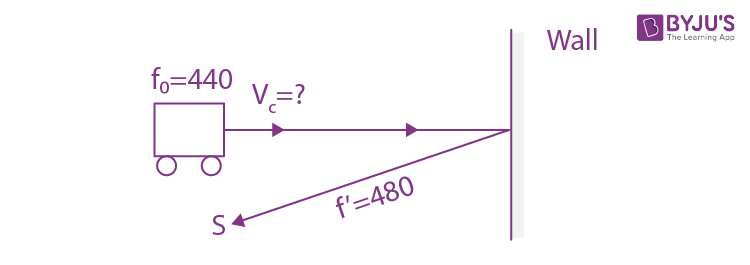480 = {[v + vc] / [v - 0]} × f1 ⇒ {[v + vc] / [v] * [v] / [v - vc]} × f0

480 = [345 + VC] * [440 / (345 - VC)]

12 = {[345 + VC] / [345 - VC]} * 11

12 × 345 – 12 × VC = 345 × 11 + 11 VC

23VC = 4200 – 3850 = 345

VC = (345 / 23) m

VC = (345 / 23) * (18 / 5) km / h

= [70 * 18] / 23

= 54.78

= 54 km/hr

Question 17: An iron rod of volume 10–3m3 and relative permeability 1000 is placed as core in a solenoid with 10 turns/cm. If a current of 0.5 A is passed through the solenoid, then the magnetic moment of the rod will be:

1. 1) 0.5 × 102 Am2
2. 2) 50 × 102 Am2
3. 3) 5 × 102 Am2
4. 4) 500 × 102 Am2

Solution:

M = μrNiA

Here μr

= Relative permeability

N = No. of turns

i = Current

A = Area of cross section

M = μrNiA = μrnℓiA

M = μrniV = 1000(1000) 0.5 (10-3)

= 500

= 5 × 102 Am2

Question 18: Two coherent sources of sound, S1 and S2, produce sound waves of the same wavelength, λ = 1 m, in phase. S1 and S2 are placed 1.5 m apart (see fig). A listener, located at L, directly in front of S2 finds that the intensity is at a minimum when he is 2 m away from S2. The listener moves away from S1, keeping his distance from S2 fixed. The adjacent maximum of intensity is observed when the listener is at a distance d from S1. Then, d is :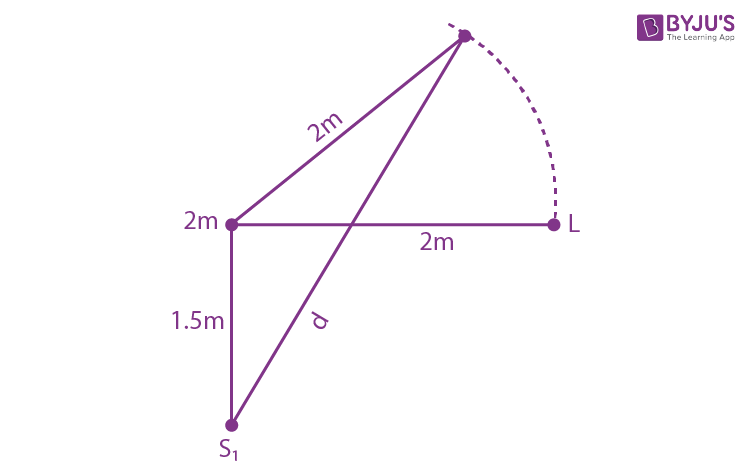1. 1) 12 m
2. 2) 2 m
3. 3) 3 m
4. 4) 5 m

Solution: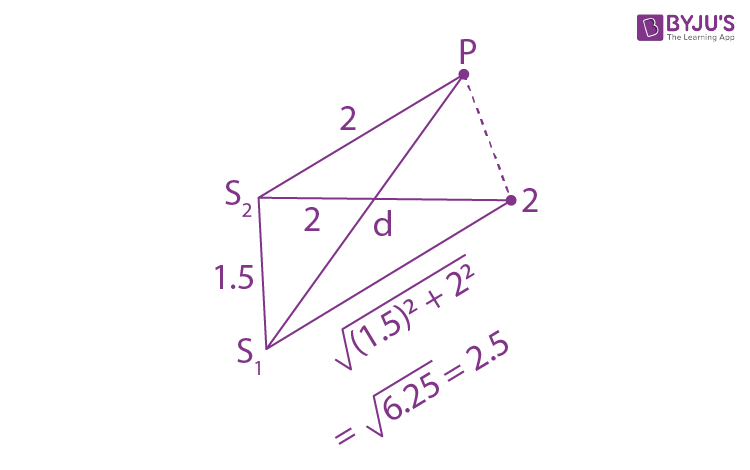For min at (L)

S1 L – S2 L = Δx = [λ / 2] (2n + 1);(n = 0, 12)

2.5 – 2 = [1 / 2] (2n + 1)

0.5 × 2 = (2n + 1)

2n = 0

n = 0 (first minima)

The adjacent maxima is the first maxima.

So at 'p' → first maxima

⇒ S1P – S2P = λ [n = 1] for first maxima

S1P – 2 = 1

S1P = 1 + 2

d = 3 m

Question 19: The quantities x = 1 / √μ0ε0, y = E / B and z = L / CR are defined where C - capacitance, R - Resistance, L - length, E - Electric field, B - magnetic field and ε0, μ0 - free space permittivity and permeability respectively. Then:

1. 1) Only y and z have the same dimension
2. 2) x, y and z have the same dimension
3. 3) Only x and y have the same dimension
4. 4) Only x and z have the same dimension

Solution:

x = 1 / √μ0ε0 = (speed)

[x] = LT-1 y = E / B = speed

Z = l / CR = m / sec = m / s [y] = LT-1

[RC = T]

[Z] = LT–1

So, x, y, z has the same dimension.

Question 20: The acceleration due to gravity on the earth’s surface at the poles is g and angular velocity of the earth about the axis passing through the pole is ω. An object is weighed at the equator and at a height h above the poles by using a spring balance. If the weights are found to be same, then h is : (h < < R, where R is the radius of the earth)

1. 1) R2ω2 / g
2. 2) R2ω2 / 8g
3. 3) R2ω2 / 4g
4. 4) R2ω2 / 2g

Solution:

∵ The weight same at poles and at h (so g1 = g2)

g1 = g – Rω2

g2 = g [1 - (2h / R)]

∵ g1 = g2

g – Rω2 = g [1 - (2h / R)]

2 = 2gh / R

h = R2ω2 / 2g

Question 21: Nitrogen gas is at 300° C temperature. The temperature (in K) at which the rms speed of a H2 molecule would be equal to the rms speed of a nitrogen molecule, is _________. (Molar mass of N2 gas 28 g).

Solution:

Vrms = √(3RT / M)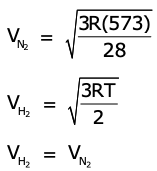√(3RT / 2) = √([3R * 573] / 28)

T / 2 = 573 / 28

T = 41 K

Question 22: The surface of a metal is illuminated alternately with photons of energies E1 = 4 eV and E2 = 2.5 eV respectively. The ratio of maximum speeds of the photoelectrons emitted in the two cases is 2. The work function of the metal in (eV) is _________.

Solution:

[(1 / 2)mV12] / [(1 / 2)mV22] = [E1 - ɸ0] / [E2 - ɸ0] = [4 - ɸ0] / [2.5 - ɸ0]

(V1 / V2)2 = [4 - ɸ0] / [2.5 - ɸ0]

(2)2 = [4 - ɸ0] / [2.5 - ɸ0]

10 - 4ɸ0 = 4 - ɸ0

0 = 10 - 4 = 6

ɸ0 = 2eV

Question 23: A prism of angle A = 1° has a refractive index μ = 1.5. A good estimate for the minimum angle of deviation (in degrees) is close to N / 10. Value of N is

Solution:

A = 1°

δ = (μ – 1) A

= (1.5 – 1) A

= 0.5 × 1

= 5 / 10 = N / 10 so N = 5

Question 24: A body of mass 2 kg is driven by an engine delivering a constant power of 1 J/s. The body starts from rest and moves in a straight line. After 9 seconds, the body has moved a distance (in m) _____________.

Solution:

P = Fv = mav

a = p / mv

dv / dt = p / mv

0u v dv = (p / m) ∫0t dt

u2 / 2 = (p / m) t

u = √(2p / m) * (√t)

dx / dt = √(2p / m) * (√t)

0x dx = √(2p / m) ∫09 √t dt

x = (2 / 3) [(9)½]3

= (2 / 3) * 27

x = 18

II-method

Pt = w = [1 / 2] mv2 – 0

1 × t = [1 / 2] × 2 × u2

u = √t

(dx / dt) = √t = ∫01 dx = ∫09 √t dt

x = [t3/2]09 / (3 / 2) = 18m

Question 25: A thin rod of mass 0.9 kg and length 1 m is suspended, at rest, from one end so that it can freely oscillate in the vertical plane. A particle of mass 0.1 kg moving in a straight line with velocity 80 m/s hits the rod at its bottom most point and sticks to it (see figure). The angular speed (in rad/s) of the rod immediately after the collision will be __________.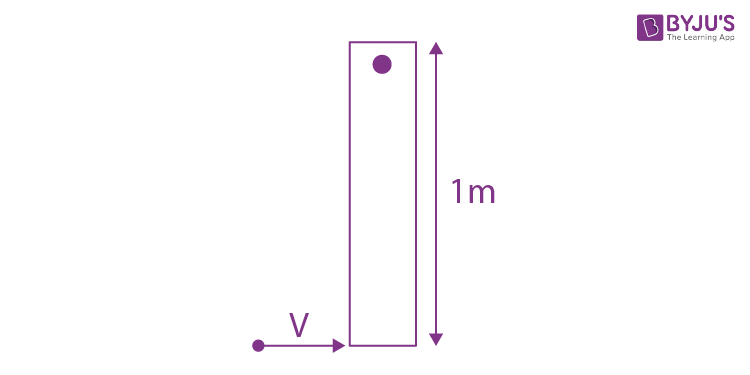Solution:

Li = Lf

0.1 × 80 × 1 = {[0.9 × 12] / 3} × ω + (0.1) 12 ω

8 = (0.3 + 0.1) ω

8 = (0.4) ω

ω = 80 / 4 = 20

### JEE Main 2020 Physics Paper With Solutions Shift 2 September 5